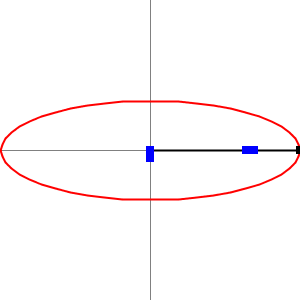# Equation of an ellipse

Téma:
Elipsa
An ellipse is a plane curve surrounding two focal points, such that for all points on the curve, the sum of the two distances to the focal points is a constant. Analytically, the equation of a standard ellipse centered at the origin with width 2a and height 2b is: Elipsa je dána středem S =(m, n) a velikostmi poloos a, b. Vyjádřete elipsu implicitně rovnicí v osovém tvaru a parametricky.
Change the value for semi-major axis a and semi-minor axis b by draging the sliders a, b.

## Parametric (vector) form of an elipse.

The equation of a standard ellipse centered at the S=(-2,2) with width 2a = 6 and height 2b = 4 is:

Zde označte odpověď
• A
• B
• C
• D

## Implicit quadratic equation of an elipse.

The equation of a standard ellipse centered at the S=(-2,2) with width 2a = 6 and height 2b = 4 is:

Zde označte odpověď
• A
• B
• C
• D

## Trammel of ArchimedesAn trammel of Archimedes consists of two shuttles which are confined ('trammelled') to perpendicular rails and a rod which is attached to the shuttles at fixed positions.